# Mathematical Ratios Can Be Solved By Nickzom Calculator – The Calculator Encyclopedia

According to Math Is Fun,

A ratio says how much of one thing there is compared to another thing.

According to Wikipedia,

A ratio may be considered as an ordered pair of numbers, as a fraction with the first number in the numerator and the second as denominator, or as the value denoted by this fraction. Ratios of counts, given by (non-zero) natural numbers, denote positive rational numbers, and as such may occasionally result in natural numbers.

According to WikiHow,

Ratios are mathematical expressions that compare two or more numbers. They can compare absolute quantities and amounts or can be used to compare portions of a larger whole. Ratios can be calculated and written in several different ways, but the principles guiding the use of ratios are universal to all.

Nickzom Calculator+ (Professional Version) – The Calculator Encyclopedia solves ratio in mathematics for any number of fractional parts.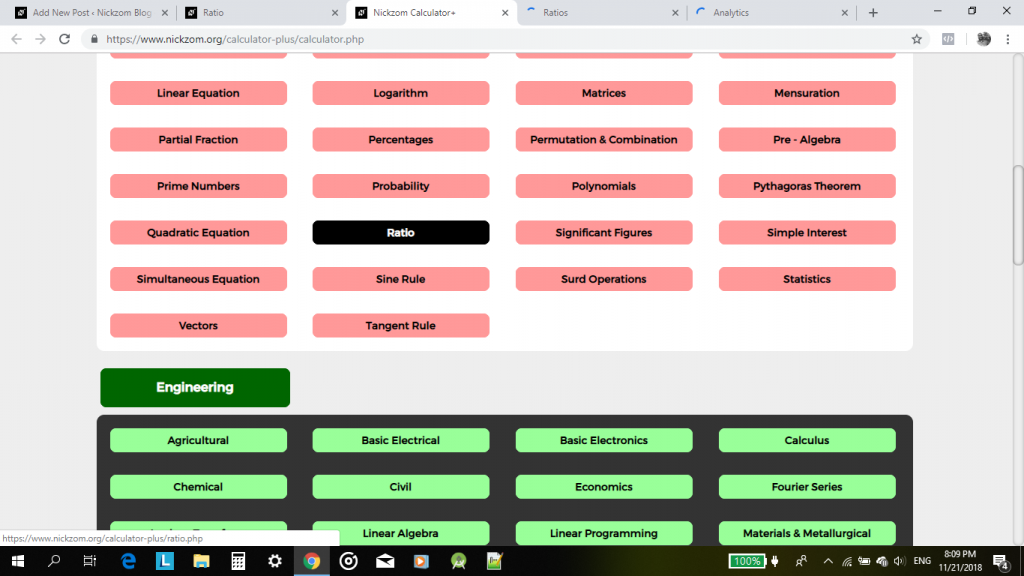For the calculator encyclopedia to be able to solve and present you a ratio. you must have to know the number of fractional parts you desire to compute the ratio for.

Now, click on Ratio on the Calculator Map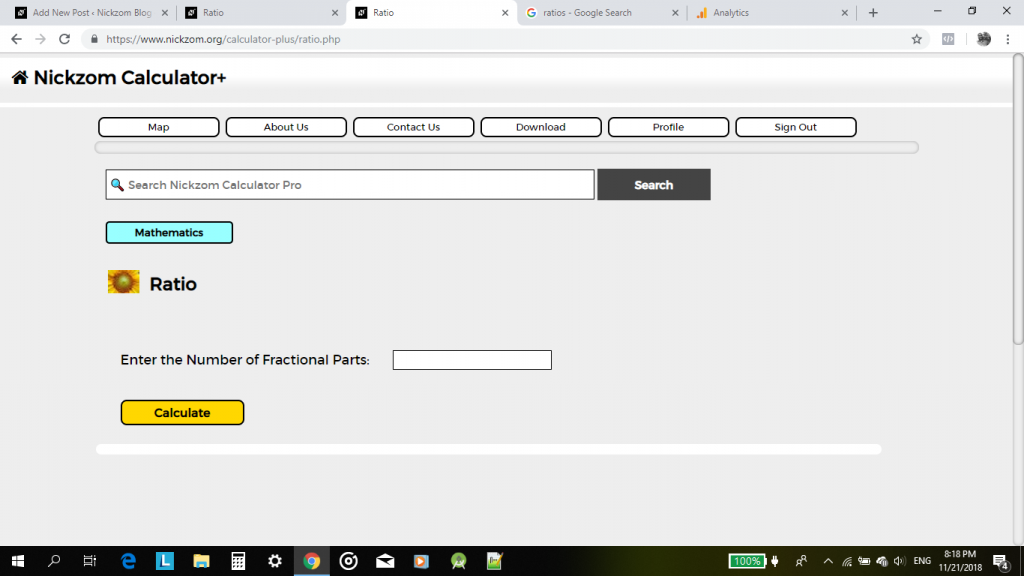For the purpose of this post, lets choose a number of fractional parts to find the ratio.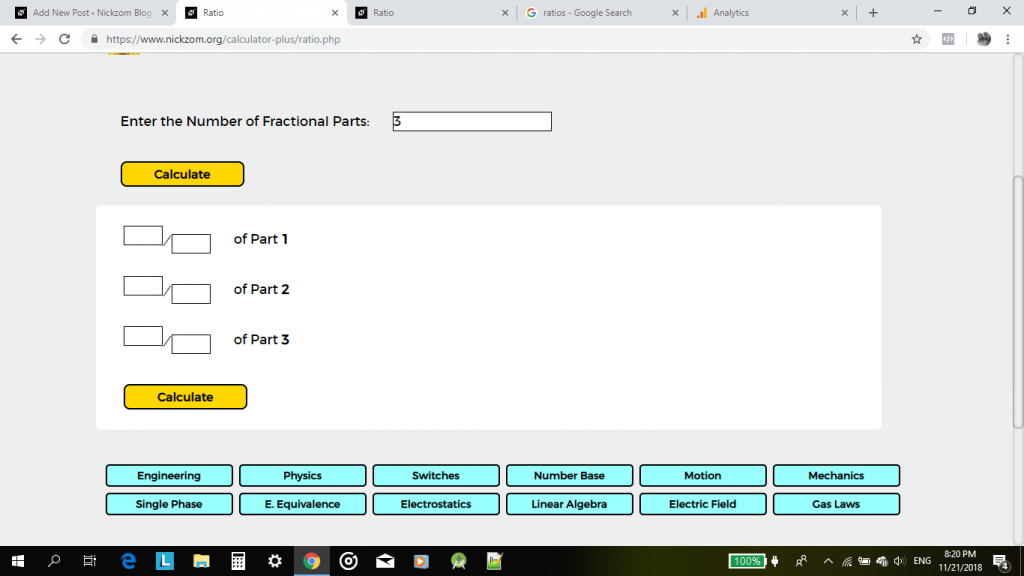Now, enter values of the fractional parts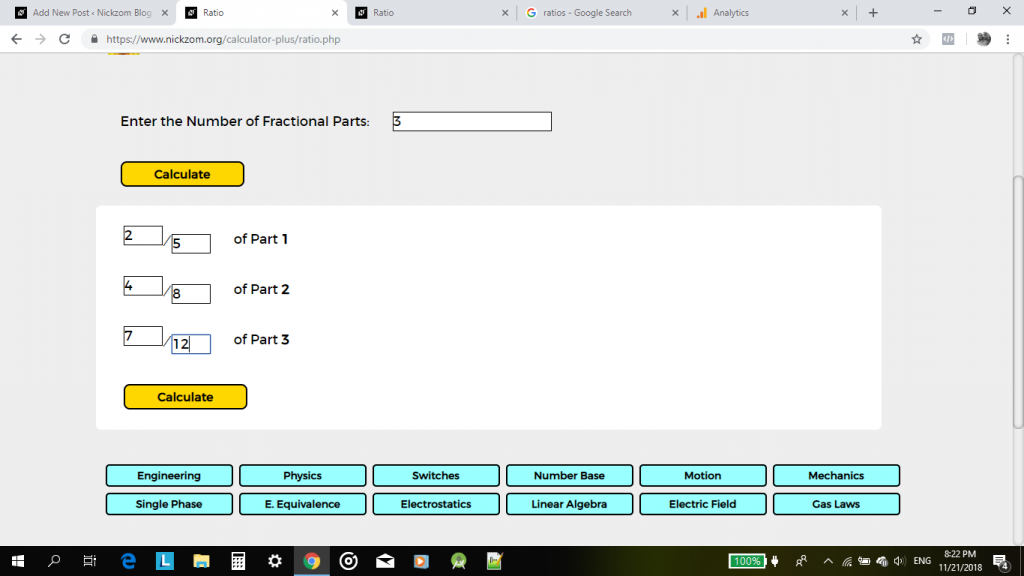Then, click on Calculate to get the result for the computation (ratio)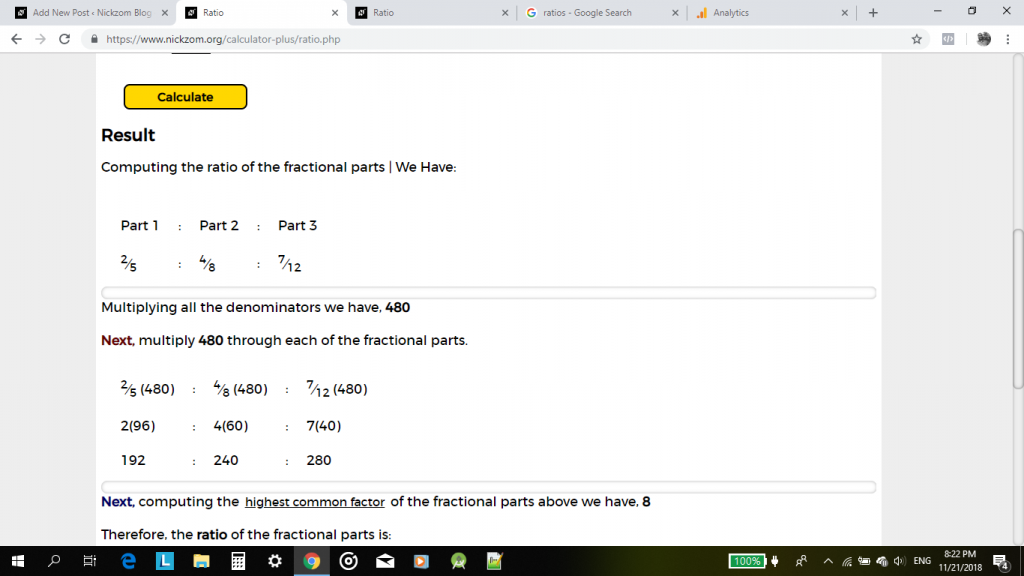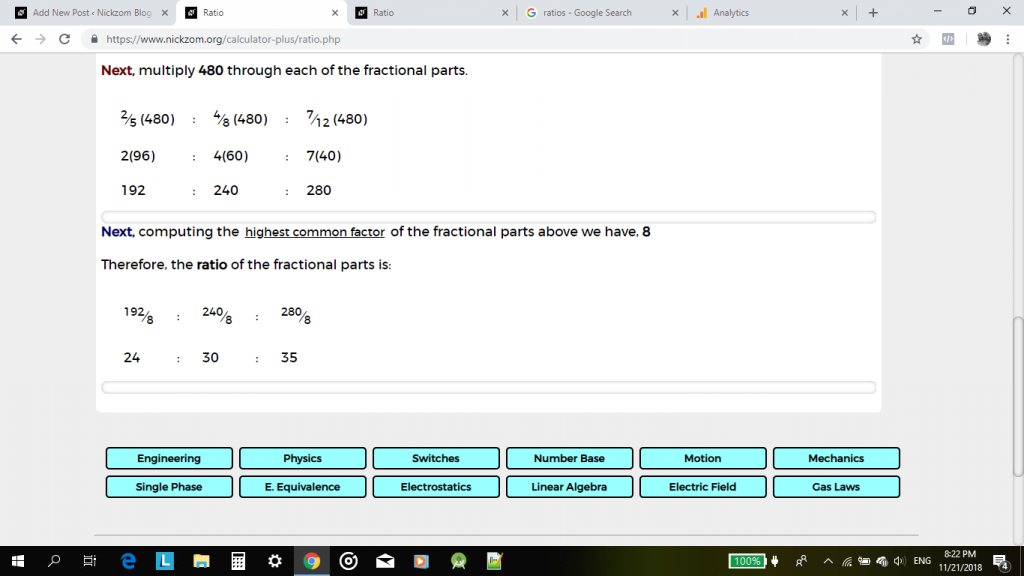One can access Nickzom Calculator+ (Professional Version) – The Calculator Encyclopedia via any of these channels: